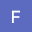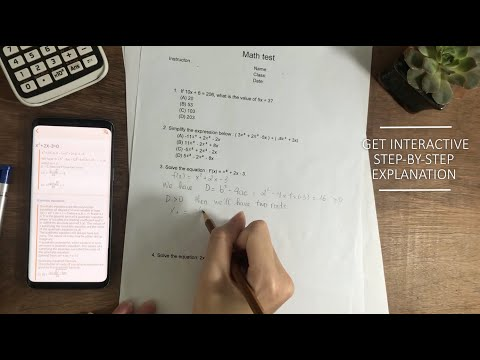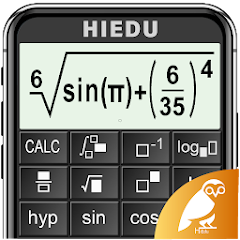# HiEdu Scientific Calculator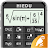4.7
84.1K reviews
5M+Everyone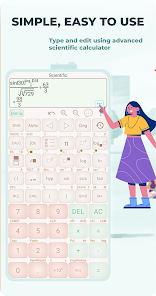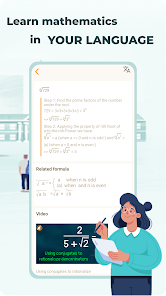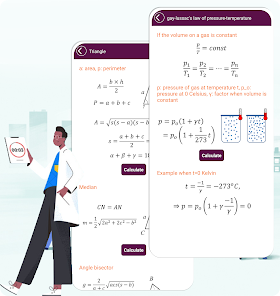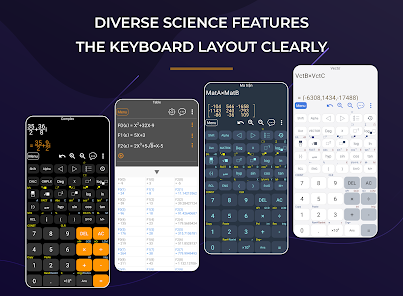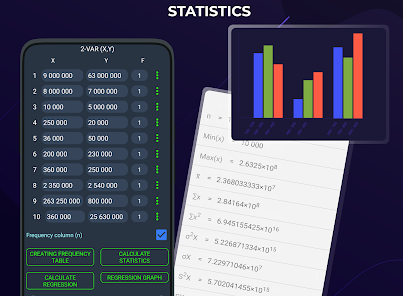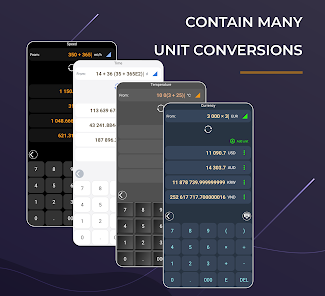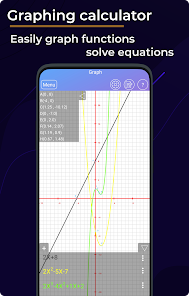HiEdu Scientific Calculator He-570: app provides help with a variety of problems including arithmetic, algebra, trigonometry, calculus, statistics, complex, matrix, vector and other topics using an advanced AI powered math solver. Simply input a problem on app. HiEdu Scientific Calculator problem solver instantly recognizes the problem and helps you to solve it with 🔥FREE Step-By-Step Explanations🔥. Quickly look up related concepts. Get help with your homework problems and gain confidence in mastering 💯 the techniques 🔥It is absolutely FREE🔥

KEY FEATURES
Scientific calculator
• It is a scientific calculator app supports most of the features of real calculators: calculator for fractions, calculator algebra, calculator derivative, calculate integral, calculate differential, calculator square root, calculator with exponents, calculator with log, calculator with remainder, calculator division, calculator with variables,.. In addition, the calculator has factorial, absolute, combination, permutation... help you calculate easily
• Natural Display makes it possible to input and display fractions and certain functions (log, x2, x3, x^), ", x−1, 10^, e^ , d/dx, Σ, Abs ...) just as they are written in your textbook
• Get interactive Step-by-Step explanation & Graphing calculator
• Save history, select a calculation in list history and edit it
• Create favorite calculations that make calculations faster

Standard calculator
• This is a perfect tool for daily calculations such as: independent memory (M+, M-), figuring percentages with memory functions similar to a small handheld calculator
• Income Tax Calculator : income tax personal, income tax calculation, sales tax calculator, tax return calculator

Complex number calculation
Complex is useful to find a complex number as a result of addition, real part of complex number, imaginary part of complex number, subtraction complex numbers calculator, multiplying complex numbers calculator and dividing complex numbers calculator

Matrix calculator
It’s main task – calculate mathematical matrices
Supported matrix operations: matrix inverse calculator, matrix determinant, matrix addition, matrix subtraction, matrix multiplication, matrix transposition

Vector calculation
This calculator app has many functions: vector calculation, calculate cross product, calculate dot product, unit vector, find angle between two vectors

Graphing calculator
• Easily graph functions such as: drawing graph, graphing calculators, find special points of functions

Solve the equation
• Solve cubic equations
• Solve inequalities
• Solve the system of equations

Converter
• Available unit conversions include:
+ Currency conversion
+ Temperature converter
+ Length converter
+ Mass/Weight
+ Speed converter
+ Area conversion
+ Cooking Volume
+ Pressure converter
+ Power converter
+ Energy
+ Time converter
+ Fuel Consumption converter
+ Digital Storage conversion

Mathematics formulas
• This app has 1000+ math formula and more to come.
• Now no need to make paper notes to remember mathematics formulas just have this app put all the formulas on your favourite phones.
• you'll find formulas very simply explained in app with necessary figures will help you to understand very easily

Physics formulas
• The application is a physical handbook. Contains most physical formulas for students and undergraduate help users quickly refer to any Physics formulas for their study and work.

Important Note : Our app products are not related to physical calculators such as casio scientific calculator, hp scientific calculators, texas scientific calculators… The app was developed by the research of the company team.
Updated on
Jan 11, 2023

## Data safety

Safety starts with understanding how developers collect and share your data. Data privacy and security practices may vary based on your use, region, and age. The developer provided this information and may update it over time.No data shared with third partiesThis app may collect these data types
Personal info, App activity and 2 others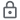Data is encrypted in transit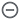Data can’t be deleted
4.7
84.1K reviews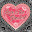MA
July 30, 2021
Highly recommend. I have 2 math 4 year degrees. Also former high school and college teacher so i have more experence than the average user. I find this calcilator app to be very useful and easy to use for both basic and more complex calculations. It has multiple features you cant find in scientific calculators. Features that are useful beyond common math courses. Before planning on use in classroom make sure your teacher checks as you may not be able to use it. Ads are not an issue.
148 people found this review helpful
HiEdu - Development scientific calculator
July 31, 2021
Thanks for your review! Please recommend our app to your friends, and don’t hesitate to shoot us a note at calculator570team@gmail.com if you have any questions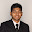Samiul Hasan
February 2, 2023
Downloaded in the middle of the class because I forgot to bring my calculator. The UI is friendly and quite similar to an actual calculator. Really finding it comfortable to use it.
11 people found this review helpful